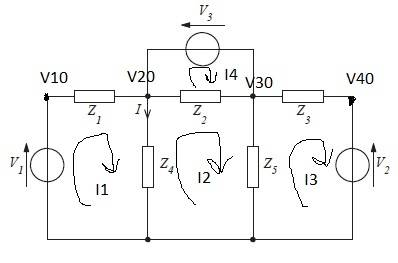# Mesh/Nodal Analysis and Matrices

Hi Everyone.

Just a brief hello before the problem! I am a new user as of today. I am studying Electrical Engineering in my spare time after work, and currently working full time an electronics service engineer. I have tried to make the problem as clear as I can, any help would be highly appreciated.

The problem asks to produce a current via two methods. I have obtained two answers that are different and would like to know where I went wrong.

For the record, most of my calculations were done within matlab. This is also my first attempt at using matrices, so I am unsure if my calculations were correct (or whether it was necessary to use matrices in the first place).

## Homework Statement

Determine the current I by

A) Mesh Analysis

B) Nodal Analysis\begin{align} &v1 = 120 < 0° V\\ &v2 = 120 < 90° V\\ &v3 = 20 < 45° V\\ &z1 = 2 Ω\\ &z2 = –j5 Ω\\ &z3 = 4 Ω\\ &z4 = –j5 Ω\\ &z5 = j4 Ω\\ \\ &Node Voltages\\ &V10\\ &V20\\ &V30\\ &V40\\ \\ &Mesh Loops\\ &I1\\ &I2\\ &I3\\ &I4\\ \end{align}

## The Attempt at a Solution

A)[/B]

I1 -
$$v1-I1(z1+z4)+I2(z4) = 0$$
$$I1(-2+j5)+I2(-j5) = -120$$

I2 -
$$I1(z4)-I2(z2+z4+z5)+I3(z5)+I4(z2) = 0$$
$$I1(-j5)+I2(j6)+I3(j4)+I4(-j5) = 0$$

I3 -
$$I2(z5)-I3(z3+z5)-v2$$
$$I2(j4)+I3(-4-j4) = j120$$

I4 -
$$I2(z2)-I4(z2)-v3$$
$$I2(-j5)+I4(j5) = 14.14+ j 14.14$$

Matrix A
$$\begin{pmatrix} -2+j5 & -j5 & 0 & 0\\ -j5 & j6 & j4 & -j5\\ 0 & j4 & -4-j4 & 0\\ 0 & -j5 & 0 & j5\\ \end{pmatrix}$$

Matrix B
$$\begin{pmatrix} -120\\ 0\\ j120\\ 14.14+j14.14\\ \end{pmatrix}$$

$$INV(A)*B = C$$

Matrix C - from matlab
$$\begin{pmatrix} 16.8112 - j22.8793\\ 25.9629 - j40.1548\\ 18.0588 - j22.0959\\ 28.7914 - j42.9832\\ \end{pmatrix}$$

$$I = I1-I2-I4$$
$$I = 16.8112 - j22.8793 - 25.9629 - j40.1548 - 28.7914 - j42.9832$$
$$I = -37.9431 + j60.2587 A$$

B)

$$V20 - V30 = V3$$

Node 2+3 supernode
$$\frac{v1-V20}{z1} - \frac{V20}{z4} - \frac{V30}{z5} + \frac{v2-V30}{z3} = 0$$
$$X = V20(-0.5 - j0.5) + V30(-0.25+j0.25) = -60-j30$$
$$(V20-V30 = v3) * (-0.25+j0.25)$$
$$Y = V20(-0.25+j0.25) + V30(0.25-j0.25) = -5\sqrt{2}$$
$$V20(-0.75+j0.05) = 67.07-j30$$
$$V20 = \frac{-67.07-j30}{-0.75+j0.05}$$
$$V20 = 86.37 + 45.76 V$$
$$I = \frac{V20}{z4} = -9.15 +j17.27$$

As you can see I have very different answers, and have scratched my head all weekend over it.
Any help would be appreciated.
Thanks!

Earthloop

gneill
Mentor
For your mesh analysis everything looks good. Your matrix is fine, your resulting mesh currents are fine. However...
When it comes time to calculate ##I##, keep in mind that mesh currents are not branch currents. Your calculation seems to be assuming that they are branch currents and you're summing (KCL) them at your node V20. This is incorrect. Two mesh currents flow through Z4. Find ##I## from them...

Thanks for the fast reply Gneill.

Now I have ...

$$I1-I4-(I2-I4) = -9.1517 + j17.2755$$

which matches my node result.

Thankyou very much!
I will be more careful in future to not mix up mesh and branch currents!

gneill
Mentor
I think you meant: simply ##I = i_1 - i_2##, since ##i_1## and ##i_2## are the mesh currents that flow in the branch where ##I## is indicated. ##i_4## doesn't enter the picture at all.

Ah.. I clearly got confused there. Thanks for clearing that up. It's been a long day.
Thanks again

The Electrician
Gold Member
Earthloop[/QUOTE]
I will be more careful in future to not mix up mesh and branch currents!

But, also note that sometimes the branch currents are the same as the mesh currents. For example, the current in branch Z1 is equal to the mesh current I1 (paying attention to the assumed direction of the currents); the current in branch Z3 is equal to the mesh current I3.

Loop analysis (as distinguished from mesh analysis) allows paths for virtual currents that may encompass several meshes. Often a loop can be chosen so that the virtual loop current is the same as a desired branch current. Had you chosen the current I2 to traverse a clockwise loop through Z1, Z2, Z5 then back along the bottom to V1 and closing at Z1 (encompassing both I1 and I2 meshes as shown o, then virtual current I1 would be equal to your desired current I, and you wouldn't have to add two mesh currents to get I.

Ok, thanks for the input

Could you give an example of the calculation? I am a visual learner, after all! ;)
So are you saying I should have treated I2 as :
$$I2(- 2 + j) + I3(j4) - I4(j5) = - 120$$

Where does I1 then come into play to give me the direct answer?

Thanks

The Electrician
Gold Member
Ok, thanks for the input

Could you give an example of the calculation? I am a visual learner, after all! ;)
So are you saying I should have treated I2 as :
$$I2(- 2 + j) + I3(j4) - I4(j5) = - 120$$

Where does I1 then come into play to give me the direct answer?

Thanks
Redo your A and B matrices using what you have just got, solve, and I1 should be equal to your required I. Give it a try; what do you get?

This works because now the only mesh current though Z4 is I1. I2 is now a loop current that doesn't pass through Z4.

This is not a mesh solution, however, it's a loop method solution.